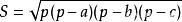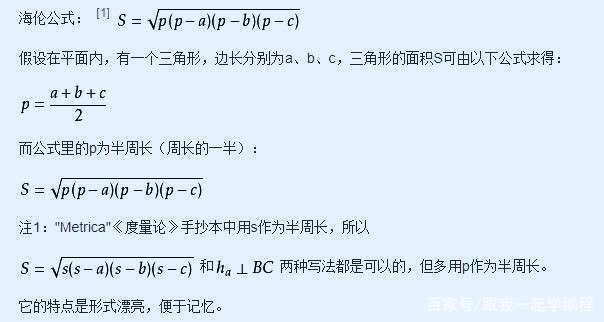• 用C#编写的一个计算器，该计算器可以根据三角形的三个边采用海伦公式，计算出每个边的高；也可以根据三棱锥（四面体）的三条斜边和三个底边，采用海伦秦九韶体积公式计算出该三棱锥（四面体）的底边面积、体积和底...
• 海伦公式的再次探究与延伸 ——关于多边形面积的计算问题 Further Exploration of The Heron's Formula ______ With
• 海伦公式计算三角形面积. 已知3个边长A,B,C. 设S=(A+B+C)/2. 面积=SQRT(S*(S-A)*(S-B)*(S-C)).算法
• 关键点： 1.注意scanf()函数的输入特点，回车符仍会留在输入缓冲区，故需注意及时清理输入缓冲区内容，避免造成输入...功能：利用海伦公式求三角形面积，若三边无法构成三角形，则面积为0 输入示例： 3 1 2 3 3 4 5 ...
关键点： 1.注意scanf()函数的输入特点，回车符仍会留在输入缓冲区，故需注意及时清理输入缓冲区内容，避免造成输入逻辑的混乱； 2.注意三角形构成条件的判定。
/*--------------------------------------------------------------
功能：利用海伦公式求三角形面积，若三边无法构成三角形，则面积为0
输入示例：
3
1 2 3
3 4 5
5 12 13
输出示例：
0.00
6.00
30.00
Author: Zhang Kaizhou
Date: 2019-3-7 11:05:04
---------------------------------------------------------------*/
#include <stdio.h>
#include <stdlib.h>
#include <math.h>

typedef struct node{
float area;
struct node * pnext;
} Node;

void compute_area(Node * p);
void list_tail_insert(Node ** pphead, Node ** pptail, Node * p);

int main(){
Node * phead = NULL, * ptail = NULL;
int n, i;
scanf("%d", &n);
getchar(); // 取出输入缓冲区的回车符
for(i = 0; i < n; i++){
Node * pnew = (Node *)calloc(1, sizeof(Node));
compute_area(pnew);
}

free(ptail);
return 0;
}

void compute_area(Node * p){
float a, b, c;
float q; //半周长
if(a + b < c || a + c < b || b + c < a || abs(a - b) > c || abs(a - c) > b || abs(b - c) > a){
p->area = 0;
}else{
q = (a + b + c) / 2;
p->area = sqrt(q * (q - a) * (q - b) * (q - c));
}
return;
}

void list_tail_insert(Node ** pphead, Node ** pptail, Node * p){
* pptail = p;
}else{
(* pptail)->pnext = p;
* pptail = p;
}
return;
}

}
return;
}


展开全文求三角形面积
• 给出了海伦公式在n维欧氏空间的推广形式,并用行列式来表示它。具体计算出用四面体的六条棱长来表示四面体的体积公式,该公式共有22项。
• 海伦公式又译作希伦公式、海龙公式、希罗公式等，它是利用三角形的三条边的边长直接求三角形面积的公式，表达式为： 其中p是三条边的和的一半儿。 python根据三角形三条边求面积 1.三角形的三条边的符合条件 ...
前言
从小学我们都知道，三角形的面积是底乘以高除以2。那么已知任意一个三角形的三条边，如何能够求出三角形的面积呢？这里我们用到了海伦公式。
海伦公式又译作希伦公式、海龙公式、希罗公式等，它是利用三角形的三条边的边长直接求三角形面积的公式，表达式为：其中p是三条边的和的一半儿。python根据三角形三条边求面积
1.三角形的三条边的符合条件
我们知道，三角形有三条边，且三条边需要满足两边之和大于第三边，否则不构成三角形。
2.海伦公式求三角形面积
a = float(input('输入三角形第一边长： '))
b = float(input('输入三角形第二边长： '))
c = float(input('输入三角形第三边长： '))
while a+b<c or a+c<b or b+c<a:
print('输入的边构不成三角形，请重新输入！')
a =float(input ('输入三角形第一边长：'))
b =float(input ('输入三角形第二边长：'))
c =float(input ('输入三角形第三边长：'))
# 计算半周长
s = (a + b + c) / 2
# 计算面积
area = (s*(s-a)*(s-b)*(s-c)) ** 0.5
print('三角形面积为 %0.2f' %area)
结语
在本例当中，我们用到了input输入，float类型转换。且根据三条构成条件使用while做循环判断，最后利用海伦公式，借助幂次运算函数完成了python的学习。在本例中**作为幂次运算是重点。
前言
在我们小时候读小学的时候就知道，三角形的面积是底乘以高除以2。那么已知任意一个三角形的三条边，如何能够求出三角形的面积呢？下面我们用到了海伦公式。
海伦公式又译作希伦公式、海龙公式、希罗公式等，它是利用三角形的三条边的边长直接求三角形面积的公式，表达式为：其中p是三条边的和的一半儿。python根据三角形三条边求面积
1.三角形的三条边的符合条件
大家都知道，三角形有三条边，且三条边需要满足两边之和大于第三边，否则不构成三角形。
2.海伦公式求三角形面积
a = float(input('输入三角形第一边长： '))
b = float(input('输入三角形第二边长： '))
c = float(input('输入三角形第三边长： '))
while a+b<c or a+c<b or b+c<a:
print('输入的边构不成三角形，请重新输入！')
a =float(input ('输入三角形第一边长：'))
b =float(input ('输入三角形第二边长：'))
c =float(input ('输入三角形第三边长：'))
# 计算半周长
s = (a + b + c) / 2
# 计算面积
area = (s*(s-a)*(s-b)*(s-c)) ** 0.5
print('三角形面积为 %0.2f' %area)
结语
在本文当中，我们用到了input输入，float类型转换。且根据三条构成条件使用while做循环判断，最后利用海伦公式，借助幂次运算函数完成了python的学习。在本例中**作为幂次运算是重点。
总结
他山之石，可以攻玉。你不努力，永远不会有人对你公平，只有你努力了，有了资源，有了话语权以后，你才可能为自己争取公平的机会。从2014年起，python一路披荆斩棘，走上神坛，而其他语言VisualBasia，c#等排名有下滑。正如《琅琊榜》中的诗句所言：“遥映人间冰雪样，暗香幽浮曲临江。遍识天下英雄路，俯首江左有梅郎”。时势造英雄，现今AI有Python。如果大家对python感兴趣，可以长按扫描下方的二维码。展开全文python
• 2012年苏州大学人机交互课程课题作业 海伦公式 输入三边计算出三角形面积 同时形成该三角形
• 海伦公式 如果有一个三角形，它的三边分别为 $a, b ,c$, 则三角形的面积为 A=s(s−a)(s−b)(s−c)A = \sqrt{s(s-a)(s-b)(s-c)}A=s(s−a)(s−b)(s−c)​ , s=a+b+c2s = \frac{a+b+c}{2}s=2a+b+c​ 二 判断一点和...
一 海伦公式
如果有一个三角形，它的三边分别为 $a, b ,c$, 则三角形的面积为

A

=

s

(

s

−

a

)

(

s

−

b

)

(

s

−

c

)

A = \sqrt{s(s-a)(s-b)(s-c)}

,

s

=

a

+

b

+

c

2

s = \frac{a+b+c}{2}

二 判断一点和三角形的关系
三角形的三点为

A

,

B

,

C

A, B, C

，我们需要判断的点是

D

D

如果

S

△

A

B

C

=

S

△

B

C

D

+

S

△

A

B

D

+

S

△

A

C

D

S\triangle ABC = S\triangle BCD + S\triangle ABD + S\triangle ACD

点

D

D

在

△

A

B

C

\triangle ABC

的内部，否则

D

D

在三角形的外部
三 example and code
洛谷模版题
#include<iostream>
#include<cmath>
using namespace std;
int s,t,u,v,w,x,y,z;
char ch;
double m(int a,int b,int c,int d,int e,int f)//海伦公式算三角形面积
{
double x,y,z,p;
x=sqrt((a-c)*(a-c)+(b-d)*(b-d));
y=sqrt((a-e)*(a-e)+(b-f)*(b-f));
z=sqrt((c-e)*(c-e)+(d-f)*(d-f));
p=(x+y+z)/2;
return sqrt(p*(p-x)*(p-y)*(p-z));
}
int main()
{
cin>>ch>>s>>ch>>t>>ch;//点A
cin>>ch>>u>>ch>>v>>ch;//点B
cin>>ch>>w>>ch>>x>>ch;//点C
cin>>ch>>y>>ch>>z>>ch;//点D
double abc,abd,acd,bcd;//几个三角形的面积
abc=(int)m(s,t,u,v,w,x)*100;
abd=(int)m(s,t,u,v,y,z)*100;
acd=(int)m(s,t,w,x,y,z)*100;
bcd=(int)m(u,v,w,x,y,z)*100;
if((s==y&&t==z)||(u==y&&v==z)||(w==y&&x==z))
{
cout<<4;    //4
return 0;
}
if(abd+acd+bcd>abc)
{
cout<<2;    //2
return 0;
}
else
{
if(!abc||!abd||!acd||!bcd)
{
cout<<3;    //判断3
return 0;
}
cout<<1;//1
}
}

展开全文三角形 数学 ACM
• #include <iostream> #include <cmath> using namespace std; int main() { double a, b, c,p,s; cout << "请输入三角形三条边长" << endl; cin >>... s = sqrt
#include <iostream>
#include <cmath>
using namespace std;
int main() {
double a, b, c,p,s;
cout << "请输入三角形三条边长" << endl;
cin >> a >> b >> c;
p = (a + b + c) / 2;
s = p * (p - a) * (p - b) * (p - c);
s = sqrt(s);
cout << "三角形面积为" << s << endl;
return 0;

}

展开全文• ## c++实现海伦公式

千次阅读 2020-02-29 17:28:16
海伦公式：p=a+b+c s=√(p(p-a)(p-b)(p-c)) 先判断是否是三角形，不是三角形输出NaN，是三角形输出面积（保留两位小数） 输入样例： 2 4.0 5.0 6.0 3 4 5 输出样例： NaN 6.00 注意：为保留两位小数，头文件加 #...
• 计算三角形面积 首先要判定输入的3条边是否能构成三角形，如果可以，根据海伦公式计算三角形面积，否则输出错误信息。 #include #include int main() { double a,b,c,p,area; pintf("请输入三角的边长(a,b,c):"); ...c语言 算法
• 如图： A D 垂 直 于 B ...\Large\therefore S_{\triangle ABC}=\frac{1}{2}ah=\frac{1}{2}a\times \frac{\large\sqrt{\normalsize4a^2b^2-\small(a^2-c^2+b^2)\large^2}}{2a}=\frac{1}{...化简即为海伦公式。 证毕
•C语言 利用三边求三角形面积 三角形面积公式
• 1.长方形面积的推导 数学家规定： 边长是一厘米的正方形面积是一平方厘米。 边长是一分米的正方形面积是一平方... 所以长方形的面积公式为: 2.平行四边形面积的推导 平行四边形能通过移动变成我们熟知的长...算法 机器学习
• 三角形三边分别为 a,b,c，三角形的半周长为p，面积为S 存在公式定理如下： 代码如下： #include #include int main() { int a=3,b=4,c=5; double p=0; double S=0; p = (a+b+c)/2; S = sqrt(p*(p-a)*(p-b)*(p-c)); ...c语言
• ")) b = float(input("请输入第二条边长：")) c = float(input("请输入第三条边长：")) #求出半周长 p = (a + b + c) / 2 #使用海伦公式计算面积 area = (p*(p-a)*(p-b)*(p-c))**0.5 #输出面积 print("面积为：%.2f...python
• 首先我们来介绍一下什么是海伦公式 海伦公式又译作希伦公式、海龙公式、希罗公式、海伦－秦九韶公式。它是利用三角形的三条边的边长直接求三角形面积的公式。表达式为：S=√p(p-a)(p-b)(p-c)，其中p等于周长的一半...c语言
• c) > b and (b + c) > a: #根据海伦公式（p=(a+b+c)/2）s=√[p(p-a)(p-b)(p-c)] p = (a + b + c) / 2 s = float(math.sqrt( p * ( p - a ) * ( p - b) * ( p - c))) print("三角形面积为:{0}".format(s)) else: ...python
• 容易想到通过三角形面积来建立等量关系，我们知道三条边a,b,c，以及内切圆的半径R，容易想到通过三角形面积来建立等量关系， 由海伦公式：由海伦公式：由海伦公式： S△ABC=p(p−a)(p−b)(p−c)，其中p=a+b+c2S_{\...计算几何 ACM 算法
• python语言利用海伦公式计算三角形面积 #计算三角形面积 #键盘接收三条边长 a=int(input("输入边长a")) b=int(input("输入边长b")) c=int(input("输入边长c")) #求半周长p p=(a+b+c)/2 #根据海伦公式求面积 s=(p...
• 利用海伦公式求三角形面积 边长abc由键盘输入，若输入的边长不能构成三角形，输出相应提示信息。海伦公式如下： 其中， #include<stdio.h> #include<math.h> int main(){ double a,b,c,s; double ...c语言
• 海伦公式：s=sqrt(p*(p-a)(p-b)(p-c))。 假设在平面内，有一个三角形，边长分别为a、b、c，三角形的面积S可由以下公式求得：s=sqrt(p*(p-a)(p-b)(p-c))。 而公式里的p为半周长（周长的一半）：p=1/2(a+b+c)。 C++...
• 代码如下： 运行结果自行输入三边长即可c语言
• 海伦公式： 1、p=(a+b+c)/2 2、面积=对p*(p-a)*(p-b)*(p-c)进行开根号 #include<stdio.h> #include<math.h> int main(){ double a,b,c,p,res; scanf("%lf%lf%lf",&a,&b,&c); p......